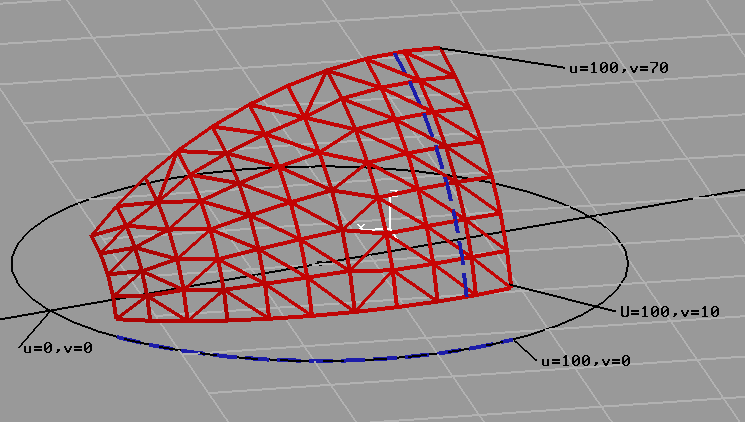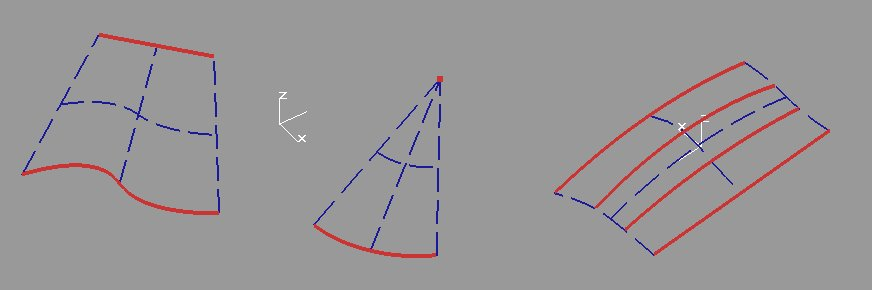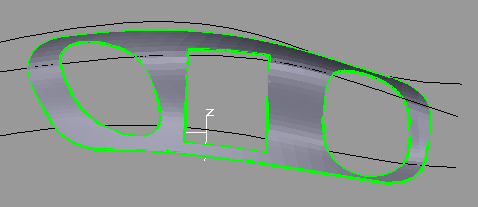```

Description format surfaces

A planar Surf. (Trim / punch)
Planar surface; unperforated or perforated

Input: boundary [islands ...]
The curves used for boundary and islands must be closed;
use circle, ellipse, closed B-Spline, closed polygon
or closed CCV.

Output:
A = boundary curve [islands ...]

# Example circular surface:
C20=P(0 0 0) 10
A20=C20

# Example Planar surface:
P20 = P (-120 -160)
# The boundary curve:
S20 = CCV P20 P (233 -186) P (223 -95) P (104 -81) P (135134) P (-122 162) P20
# The planar surface:
A20 = S20

# Example: outer edge C29, island C28.
C29 = P (-252.2 -580.9 0) VAL (12)
C28 = P (-255.9 -606.8 0) VAL (66)
A20 = C28 C29

A spheric.Surf (Axis, wheel) SPH
Spherical surface

Format:
A = SPH axis radius [drehWinkel1 drehWinkel2 [HoeheWinkel1 HoeheWinkel2]]
Axis:      The main axis of the ball
rotAngle1  KeyIn angle (Def = 0) or sel. point.
rotAngle2  KeyIn angle (Def = 360) or sel. point.
tiltAngle1 KeyIn angle (Def = 0) or sel. point.
tiltAngle2 KeyIn angle (Def = 180) or sel. point.# Example:
P20 = P (68 68.9 0)
A21 = R SPH (P20) 12

Cylindrical surface

Format:
A = CYL axis radius rotAngle1 rotAngle2 height1 height2
Axis:     The main axis of the cylinder; line, vector or plane (z-axis).
rotAngle1 KeyIn launch angle (Def = 0) or sel point.
rotAngle2 KeyIn End piece (Def = 360) or sel point.
height1   KeyIn elevation (from Achsstartpunkt; Def = 0) or sel point.
height2   KeyIn elevation (from Achsstartpunkt) or sel point.

# Examples:
L1=P(0 0) P(100 0)
A1=CYL L1 12 0 360 0 30

R2=PERP P(105 129.2 0) D(1 1 1)
A2=CYL R2 12 0 180 0 30

A Revolved S. (Axis Contour) SRV
Revolved-Surface
Rotate contour-object around axis
Contour is roughly paralllel to the axis (normal: Sweep-surface)

Format:
A = SRV axis contour [Start1 End1 [Start2 End2][CW]]
Axis        Line or Plane or PT+PT or PT+LN or PT+VC
Circle: axis from centerpoint and normalvector
Contour     line (cone), circle (torus) or B-Spline.
Start1      Startpoint revolved-surface: angle (Def = 0) or point.
End1        Endpoint revolved-surface: angle (Def = 360) or point.
Start2      Startpoint contour: parameter (Def = 0) or point.
End2        Endpoint contour: parameter (Def = 1) or point.
CW          sense of rotaion of revolved-surface: def =CCW
(counterclockwise); CW is clockwise.Examples:
# cone:
L20 = P (20 0) P (20 20)
L21 = P (50 0) P (40 20)
A20 = SRV L20 L21 270 0

# torus:
P20=P(-30 -10 0)
P22=P20 X(100)
C20=P22 20 DY
P23=P20 Y(100)
P24=P20 Y(-100)
A20=SRV L(P20 DZ) C20 P22 P23 #CW

A Extrusion Surf (Contour Vec)           SRU
Extrusion surface from basic element and vector:
Basic Element: LN / AC / CV
Vector:        vector or PT-PT or LN ..# Example:
L30 = P (0 -10 20) P (0 10 20)
D30 = D (10 10 60)
A30 = SRU L30 D30

A Sweep Surf (Contour Path)              SWP

Surface from path (spine) and contour;
eg pipe from moving a circle (contour) along a line (path).
The contour always is on a plane normal to the path.
With heavy curvature of the spinecurve overcuts are possible.

Contour:       circle, ellipse, polygon.
Path:          line, circle, ellipse, spline.# Example:
P20=P(30, -4280, 0)
P21=P20 Z(2000)
L20=P20 P21
P23=P(900 -3177 0)
S21=ELL P20 P23 P(-142 -3537 0)
S20=POL P20 P(645 -5272 0) P23 P20
A20=SWP S20 L20
A21=SWP S21 L20

A Loft Surf (Curves across)              BSP
Free-form area from cross section curves.

Curves across [BSP]
Select (at least 2) cross section curves
The first and the last curve are the boundaries.
Curves can be lines, circles or b-spline-curves.
The first and/or last inputobject may be a point.
Continue with tab-key.
Finish with Enter-key.

With the function "S BSpline < join obj's" you can join
pieces to one connected cross-section-curve.# Example:
L20=P(-100 60 0) P(-100 30 0)
L21=P(-50 60 0) P(-60 80 0)
C20=ARC1 P(-20 100 0) P(0 90 10) P(20 80 0)
P20=P(-130 40 0)
P21=P(30 120 0)
#
A20=BSP U(P20 L20)
A21=BSP U(L20 L21)
A22=BSP U(L21 C20)
A23=BSP U(P21 C20)

Example models:
Sample_area_bsp0
Sample_area_bsp4
Sample_area_bsp6

A Loft Surf (Curves along/across)        BSP
Free-form area of horizontal and vertical sectioncurves.
The first and the last curve are the boundaries.
All length-wise and cross-wise curves must be a network.
Inputcurves can be points, lines, circles, polygons,  b-spline-curves.
(currently not CCV-composite curves).

If the horizontal sectioncurves meet in a point,
this point can be selected as first or last vertical curve.

Curves along [BSP]
Selectieren (at least 2) length-wise curves
Continue with tab-key.

Curves across [BSP]
Select (at least 2) cross-wise curves
Continue with tab-key.
Finish with Enter-key.Example (2 curves along; (Spline S20, Line L20);
3 curves across (Point L20, Line L21);
The curves-along form a tip in point P20.
Die Längskurven bilden im Punkt L20 eine Spitze.

P20=P(-56 -18 0)
P21=P(25 4 0)
P23=P21 Z(4)
L20=P20 P23
L21=P21 P23
S20=BSP P20 P(-30 -20 0) P(1 -11 0) P21
A20=BSP U(S20 L20) U(P20 L21)

Sample models:
Sample_area_bsp3 (5 x 4 curves)
Sample_area_bsp5 (2 x 1 edge curves)
Sample_area_bsp7 (2 x 2 boundary curves)

A trimmed supported punched FSUB
Space at Support area limited, perforated or unperforated.

Format:
A = FSUB Support-area boundary-curve [islands ...]
Support-area: Spherical, cylindrical, revolved, Extrusion or loft surface
or cone or torus;
boundary-curve, Islands:
circle, ellipse, Spline, polygon or contour (CCV).
boundary-curve and islands must be closed.

The boundary curve must be based on the Support area.
If the outer contour of the support-area is identcal with the boundary-curve,
then the name of the support-area can be used for the boundary-curve.A = FSUB A S                support-area, trimmed
A = FSUB A S S              support-area, trimmed, 1 hole
A = FSUB A A S S            support-area, not trimmed, 2 holes

A = FSUB B S                cone or Torus, trimmed
A = FSUB B S S              trimmed, perforated
A = FSUB B B S              not trimmed, perforated

Examples:
# cone surface:
P20 = P (100 0 0)
P21 = P (200 0 0)
P22 = P20 Y (120)
P23 = P21 Y (60)
P24 = P20 P22 ANG (135) DX
P25 = P21 P23 ANG (135) dx
C20 = ARC P23 P25 P21 dx
C21 = ARC P22 P24 P20 dx
# The body:
B20 = CON C21 C20
# The boundary curve:
S20 = CCV P23 P22 C21 P24 P25 C20 P23
# The cone surface:
A20 = FSUB B20 S20

P26=P(250 -750 0)
S21=REC P26 D(500 0 0) D(0 200 0)
A21=S21
P27=P(510 -660 0)
C22=P27 40
C23=P27 80
A22=FSUB A21 C23 C22

A Hatch / hatch         HAT
Hatched surface

Format:
A # = HAT boundary-curve interval direction
boundary-curve    circle, ellipse, Spline, polygon or contour.
boundary-curve must be closed.
interval          distance of the hatch lines
direction         angle of the hatch lines in degrees

Example:
P20 = P (-500 500)
S21 = CCV P20 P (400900) P (400300) P20
A20 = HAT S21 VAL (50) VAL (45)

Currently, no import / export function for hatching.
Currently no function for perforated hatches.

```